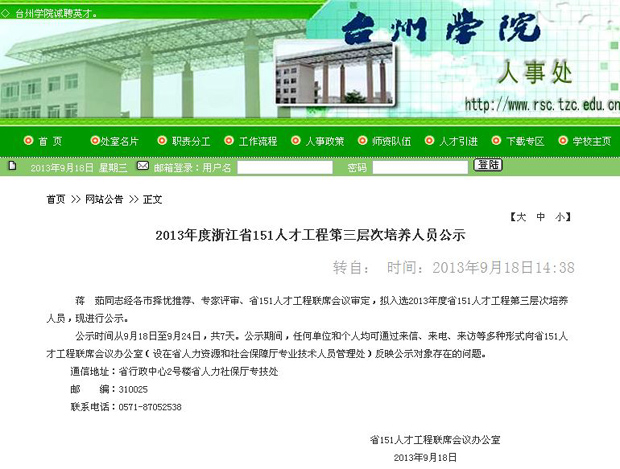• 通信地址：浙江省台州市椒江区市府大道1139号D楼246科研室
• 邮编:318000
• 电话:0576-87523645
• 传真:
• Email:zhuhuayue@126.comBioresour Technol (IF=4.98)J Hazard Mater (IF=4.14)Sep Purif Technol (IF=3.258)Chem Eng J (IF=3.07)Desalination(IF=3.04)J Environ Manag (IF=2.596)Appl Clay Sci (IF=2.342)Appl Surf Sci (IF=2.112)Ecotox Environ Saf (IF=2.202)Ceram Int (IF=1.789)Chem Eng Technol (IF=1.366)  等国外重要刊物和国际学术会上发表SCI论文30篇，EI论文10篇，ISTP论文6 篇，其中1区、2SCI论文12。发表论文被Prog Polym Sci (IF=26.383)Energ Environ Sci (IF= 11.653)Nanoscale (IF=6.233)Appl Catal B (IF=5.825)Biomacromolecules (IF=5.371)J Mater Chem (IF=5.10)Crit Rev Microbiol (IF= 5.065)(IF=4.98)Cryst Growth Des (IF= 4.689)Phys Lett B (IF=4.569)Langmuir (IF=4.27)J Membr Sci (IF=4.093)(IF=3.879)Dalton Trans (IF=3.806)Electrochim Acta (IF=3.777)Nanotechnol (IF=3.64)Appl Catal A-Gen (IF=3.64)Colloids Surf B (IF= 3.554)Sens Actuators B (IF=3.535)Dyes Pigments (IF=3.532)Carbohyd Polym (IF=3.479)Cellul(IF=3.476)Microchimica Acta (IF=3.434)Polym (IF=3.379)J A (IF=3.187)J Colloid Interface Sci (IF=3.172)Desalination (IF=3.041)国际著名SCI期刊引用超过600次，6篇论文被引用次数超过40次，单篇最高被引892SCI论文入选Web of Science近十年Highly Cited Papers”论文(工程领域引用率国际排名1％以内)。现为Bioresour Technol (IF=4.36)J Hazard Mater (IF=3.72)Chem Eng J (IF=3.07)20多个国际SCI期刊的特约审稿专家。5项科研成果获市优秀自然科学论文三等奖(2010)和校优秀科研成果一等奖(2011)、二等奖(20102012)等奖项。